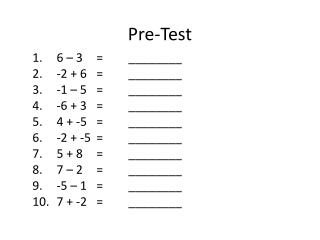DownloadDownload PresentationPre-Test

# Pre-Test

Télécharger la présentation## Pre-Test

- - - - - - - - - - - - - - - - - - - - - - - - - - - E N D - - - - - - - - - - - - - - - - - - - - - - - - - - -
##### Presentation Transcript

1. Pre-Test 6 – 3 = ________ -2 + 6 = ________ -1 – 5 = ________ -6 + 3 = ________ 4 + -5 = ________ -2 + -5 = ________ 5 + 8 = ________ 7 – 2 = ________ -5 – 1 = ________ 7 + -2 = ________

2. Learning Theory

3. What is 25 X 25 ?

4. What is 25 X 25 ?

5. Types of Learning • Problem solving (Rules) • Facts • Physical skills

6. Problem Solving • The behavior • The rule statement

7. Problem SolvingAdding Integers • The behavior-7 + 5 • The rule statementwhen the signs are different, subtract and use the sign of the larger number

8. Types of Learning • Tying your shoes • Solving: 5X + 3 = 2X – 7 • Spelling hippopotamus • Swinging a bat • knitting Name some other skills that are problem solving Name some other skills that are facts Name some other shills that are physical

9. Learning Learning is an internal process under the control of the individual Teaching is an external process under the control of the instructor

10. Students • Listen • Study • Practice

11. Teachers • Teachers set the (external prompts for the) CONDITIONS OF LEARNING

12. Conditions of Learning • For each type of learning, there should be different types of conditions

13. Conditions (Steps) in LearningProblem Solving (Original Learning) • Discriminate between items • Classify items into groups • Name the groups • Determine a solution strategy (an algorithm) • If possible, give the behavior a rule statement

14. Algorithm • A step by step procedure to solve a problem

15. Discriminate Between Items

16. Discriminate Between Items • X2 + 3X = 6 • 3X – 8 = 2X + 5 • 3X2 = 9 • 3X + 6 = 2X • 9X = 18X2 • X = 0 • 9X + X2 = 0 • How do you solve Algebra I problems? • How do you solve Algebra 2 problems?

17. A General ApproachTo Solve Problems (After Original Learning) • Recognize the problem • Remember the algorithm

18. Recognize the problemRemember the algorithm a • 8 – 3 = ________ • -3 + 5 = ________ • -4 – 9 = ________ • -4 + 5 = ________ • 8 + -5 = ________ • -3 + -6 = ________ • 5 + 4 = ________ • 3 – 2 = ________ • -6 – 2 = ________ • 9 + -5 = ________

19. Summary Types of learning Problem solving the behavior the rule statement Facts Physical skills Steps in learning (Original learning – learning for the first time) Discriminate between items Classify items into groups Name the groups Approach to Problem Solving (After original learning) Recognize the problem Remember the algorithm

20. Name the Command Structures • Tree Commands • Fluid User Interface (FUI)

21. Classify into Groups       

22. Name the Groups • Group 1 • Group 2 • Group 3